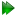The modelling of dispersion data

For a system under the influence of chemical exchange, the evolution of the transverse magnetisation is given by the Bloch (1946) equations as modified by McConnell (1958) for chemical exchange - the Bloch-McConnell equations. For a two state exchange jumping between states A and B, the equation is:=. (11.1)

The analytic or closed-form frequency-domain solution for this equation however remains intractable. Solutions can nevertheless be found by either making assumptions or restrictions about the exchange process and then analytically solving 11.1 or by finding numeric solutions. The modelling of relaxation dispersion data can hence be categorised into these two distinct methodologies:

Analytical models:
Optimisation of models based on analytical, closed-form expressions derived from the Bloch-McConnell equations subject to certain conditions (see Section 11.3 on pageand Section 11.7 on page).
Numerical models:
Optimisation of models based on numerically solving the Bloch-McConnell equations (see Section 11.4 on pageand Section 11.8 on page).

The relax user manual (PDF), created 2020-08-26.